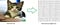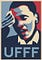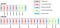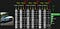Last story we talked about ordinary neural networks which are basic building blocks for deep learning, This story I wanna talk about Convolutional neural networks or Convnets.

The convnets have been the major breakthroughs in the field of Deep learning and they perform really well for image recognition, we can also use CNN’s for Natural language processing and speech analysis. In this story I focus on computer vision(Image recognition). Let’s get started!!!!!!

I follow Simon Sinek , he says always start with Why,how and finally What

Why CNN’s ??? Over Ordinary neural networks ??

Let’s say we are training a classifier to identify a cat using an ordinary neural net(where we have input, hidden and output layers)

An ordinary neural networks typically takes features as inputs, for this problem we take image array as inputs, so we have a vector, size of (image width*height) as an input.Note: I took just 10*10 grid here for understanding, but the image array size = Width*Height*Color.

We feed it to the model and train it (back propagation) for many images for many iterations.

Once the network is trained then we can give another cat picture to predict (to get the score) to see if it gives the result as cat(high probability score).

well, it works, but wait..

what if I gave the test pictures like these for prediction.

The ordinary network may not predict well(or not get much score for the cat) and what if I gave b/w pictures as test images(assume the train set does not have b/w images)

The network might fail to give the highest probability score as this type of features(b/w) we did not train.

So what is happening here??

What we feed is what we get.

The network understands the mapping between X and y but not the patterns in X.

For above 3 test images the CNN is gonna be able to predict well for cats.

ConvNets are used mainly to look for patterns in an image, we don’t need to give features, the CNN understands the right features by itself as it goes deep.this is one of the reasons why we need CNN’s. Period.

And another reason is, ordinary neural networks don’t scale well for full sized images , let’s say that input images size =100(width) * 100 (height) * 3 (rgb).

then we need to have 30,000 neurons which is very expensive in the network.

Hence We need to learn CNN.

Okay so how does it work???

For every image , it creates many images by applying some filters ( just like photo editing tools )

These filters, we can call weights , kernels or features

they are initialized randomly first then during the training these weights will get updated (the network learns these weights)

let’s take an example, suppose we have an image of 5X5 size like this and filters are like this,

Note : For sake of understanding I assume that 5 X 5 array is full image and the values are pixel values, otherwise it would be a big table of matrix and the values can be anything 0 and 1 or continuous value (- to +).

We have 3 filters which we initialize randomly (we define the filter size).

Note: Here I took 0 or 1 to make the math easy , usually these are continuous values.

if we run each filter all over the image we will get the output images like these.

How did we get the output like these???

Here is how

This is for only one filter, we take a local receptive field in the image and we apply the dot product to a scalar value then we move the window by the Stride and repeat the same process for the entire image.

This process is called Convolution.

so Step1 : Apply Convolution to all the filters for the input image.

Step 2 : Apply Pooling concept for the generate output images

for example, for the first image,

The main goal of pooling is to reduce the size of an image by taking the max values in the window, and padding is not necessary here, but for Padding explanation purpose only I added here.

Step 3 : Normalization, this is the step when you apply an activation function, the most used function here is ReLu (Rectified linear unit)

A rectified linear unit has output 0 if the input is less than 0, and raw output otherwise. That is, if the input is greater than 0, the output is equal to the input.

Here we don’t have any negative values so don’t need to apply , if we assume we have, then it will be,

Step 4 : Feed these values to Fully Connected Neural network(we talked in the last story)

This process I already covered before here, so I don’t talk about it now.

we train the model for all the images in the training set for certain no of epochs, and during training we update the weights using back propagation.

so This is how it works.Love you man!

So now what is a Convolutional neural network????

A Convolution neural network is a network of different types of layers sequentially connected together.

Types of layers

1. Convolution layer where the convolution process happens.
2. Pooling layer where the pooling process happens.
3. Normalization layer where the activation (ReLu) process happens.
4. Fully Connected layer (Dense)

A CNN can typically have multiple Convolution, pooling, Normalization layers and not necessarily following the order.

Here are the exampleshirokatsukataoka.net(Picture)Cs231n stanford.edu(Picture)

Okay now let’s make that cat follow all the steps, we will see how it looks like.

after one layer of convolution and pooling, it will look like this

I hope you get the idea how an image transforms and goes to network for training.

Here I defined 3 X 3 filter size with 3 filters and pooling size = 2 X 2

Anyway coding is not in scope in this story.

We need to define the network architecture and some parameters, then we train the model for all the images in the training set for certain no of epochs, and during training we update the weights using back propagation.

Once the training is done , just like ordinary neural networks we feed forward the test image into the network, at the end we get the probabilities scores.

Cool! right?

That’s it for this story , In the next story I will build the convolution neural network from scratch/tensorflow/keras/caffe using the above steps.

Until then

See ya!

Written by

## Deep Math Machine learning.ai

#### This is all about machine learning and deep learning (Topics cover Math,Theory and Programming)

Welcome to a place where words matter. On Medium, smart voices and original ideas take center stage - with no ads in sight. Watch
Follow all the topics you care about, and we’ll deliver the best stories for you to your homepage and inbox. Explore
Get unlimited access to the best stories on Medium — and support writers while you’re at it. Just \$5/month. Upgrade